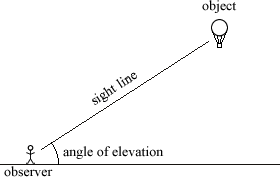index: click on a letter A B C D E F G H I J K L M N O P Q R S T U V W X Y Z A to Z index index: subject areas numbers & symbols sets, logic, proofs geometry algebra trigonometry advanced algebra & pre-calculus calculus advanced topics probability & statistics real world applications multimedia entrieswww.mathwords.com about mathwords website feedback

 Angle of Elevation The angle above horizontal that an observer must look to see an object that is higher than the observer. Note: The angle of elevation is congruent to the angle of depression (this assumes the object is close enough to the observer so that the horizontals for the observer and the object are effectively parallel; this would not be the case for a ground tracking station observing a satellite in orbit around the earth).